StatLect

Joint probability density function

The joint probability density function (joint pdf) is a function used to characterize the probability distribution of a continuous random vector.

It is a multivariate generalization of the probability density function (pdf), which characterizes the distribution of a continuous random variable.

The generalization works as follows:

• the integral of the density of a continuous variable over an interval is equal to the probability that the variable will belong to that interval;

• the multiple integral of the joint density of a continuous random vector over a given set is equal to the probability that the random vector will belong to that set.Definition

The following is a formal definition.

Definition Letbe acontinuous random vector. The joint probability density function ofis a function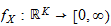such that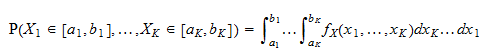for any hyper-rectangleThe notation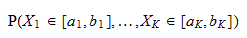used in the definition above has the following meaning:

1. the first entry of the vectorbelongs to the interval;

2. the second entry of the vectorbelongs to the interval;

3. and so on.

Furthermore, the notationwhereis a-dimensional vector is used interchangeably with the notation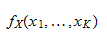whereare theentries of.

Finally, the notationmeans that the multiple integral is computed along all theco-ordinates.

Examples

Here are some examples.

Example 1

Letbe arandom vector having joint pdf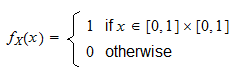In other words, the joint pdf is equal toif both components of the vector belong to the intervaland it is equal tootherwise.

Suppose we need to compute the probability that both components will be less than or equal to. This probability can be computed as a double integral: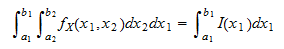Example 2

Letbe arandom vector having joint probability density function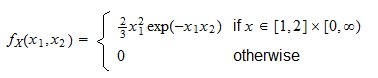Suppose we want to calculate the probability thatis greater than or equal toand at the same timeis less than or equal to.

This can be accomplished as follows: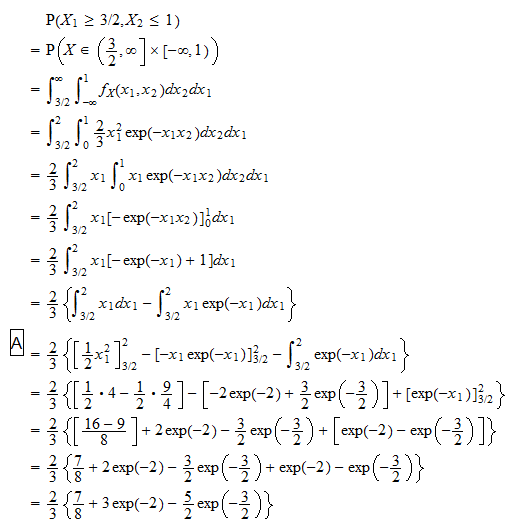where in stepwe have performed an integration by parts.

Joint and marginal density

One of the entries of a continuous random vector, when considered in isolation, can be described by its probability density function, which is called marginal density.

The joint density can be used to derive the marginal density. How to do this is explained in the glossary entry about the marginal density function.

More details

Joint probability density functions are discussed in more detail in the lecture entitled Random vectors.